Courses
Courses for Kids
Free study material
Offline Centres
More

# Easy Steps to Convert Fractions into DecimalsLast updated date: 03rd Dec 2023
Total views: 142.2k
Views today: 4.42k## Learn Fractions and Decimals with Real-life Examples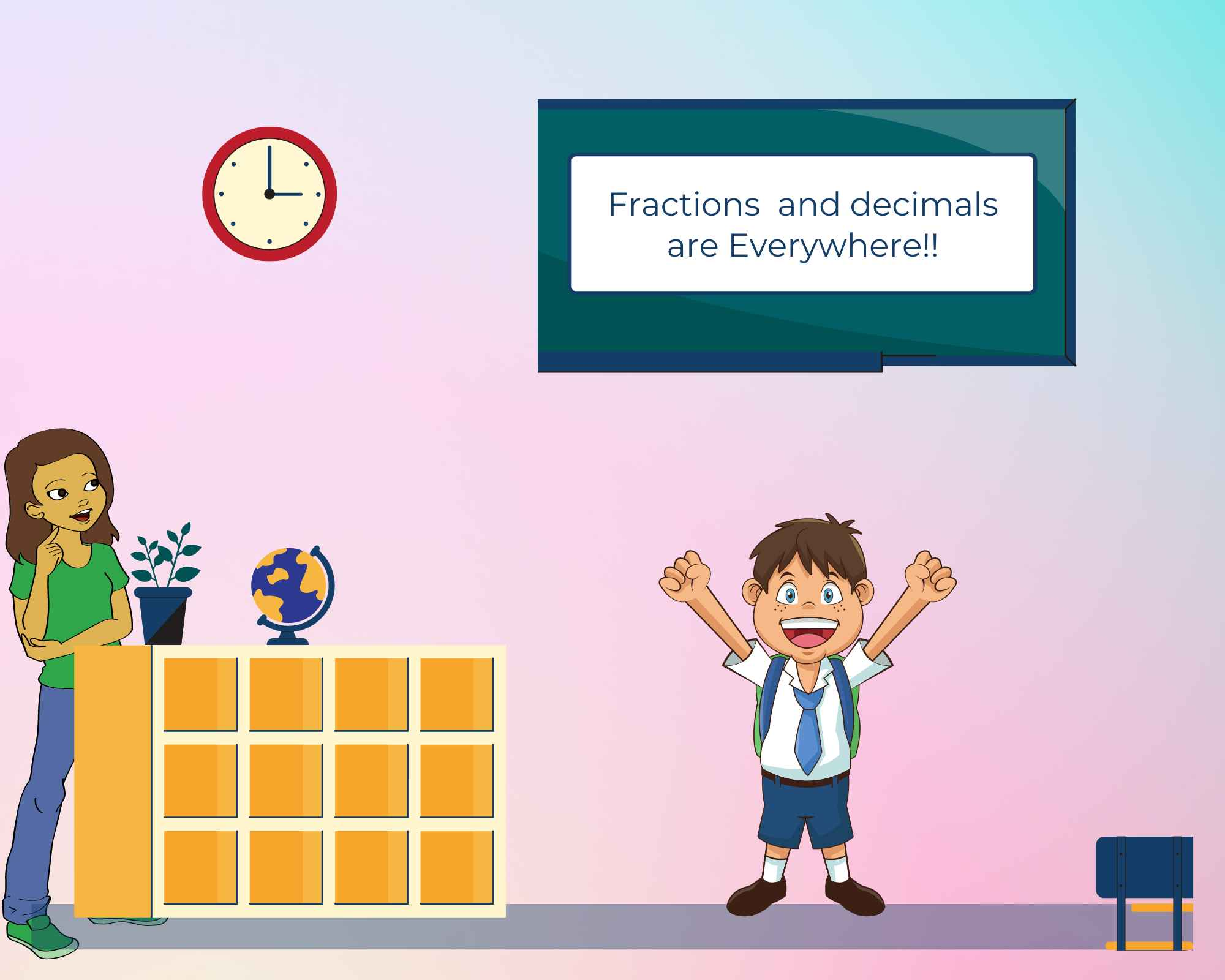Image Shows that Learning Fractions and Decimals is Fun

Any number represented as a fraction is divided into two parts: the numerator and the denominator. In general, we divide the numerator by the denominator to convert a number from fraction to decimal form. Fractions are written as p/q, where q is not equal to zero. For example, A fraction can be compared to a pizza that has been cut into equal pieces. If a pizza is cut into 10 equal parts and 4 of them have been eaten, there are 6 parts remaining. We will say that 6 out of 10 parts are still available. Comparing fractions and decimals is a little difficult. Because both fractions and decimal numbers represent the same numbers, but they don't look the same.

## Fractions

Fractions are a common way to represent partial numbers. A fraction is typically defined as a number of equal parts, such as "one half" and "two thirds." This is expressed as one whole number divided by another whole number. "Two-thirds" is written as $\frac{1}{3}$

A fraction is a part of a whole number. A fraction is a ratio of the upper number (numerator) to the lower number (denominator). The numbers are vertically kept and separated by a bar.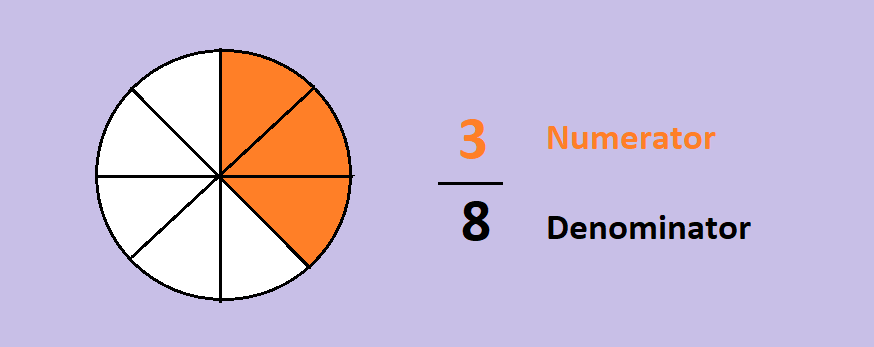The Above Image Shows the Parts of a Fraction

## Types of Fractions

Refer to the images below for different types of Fractions: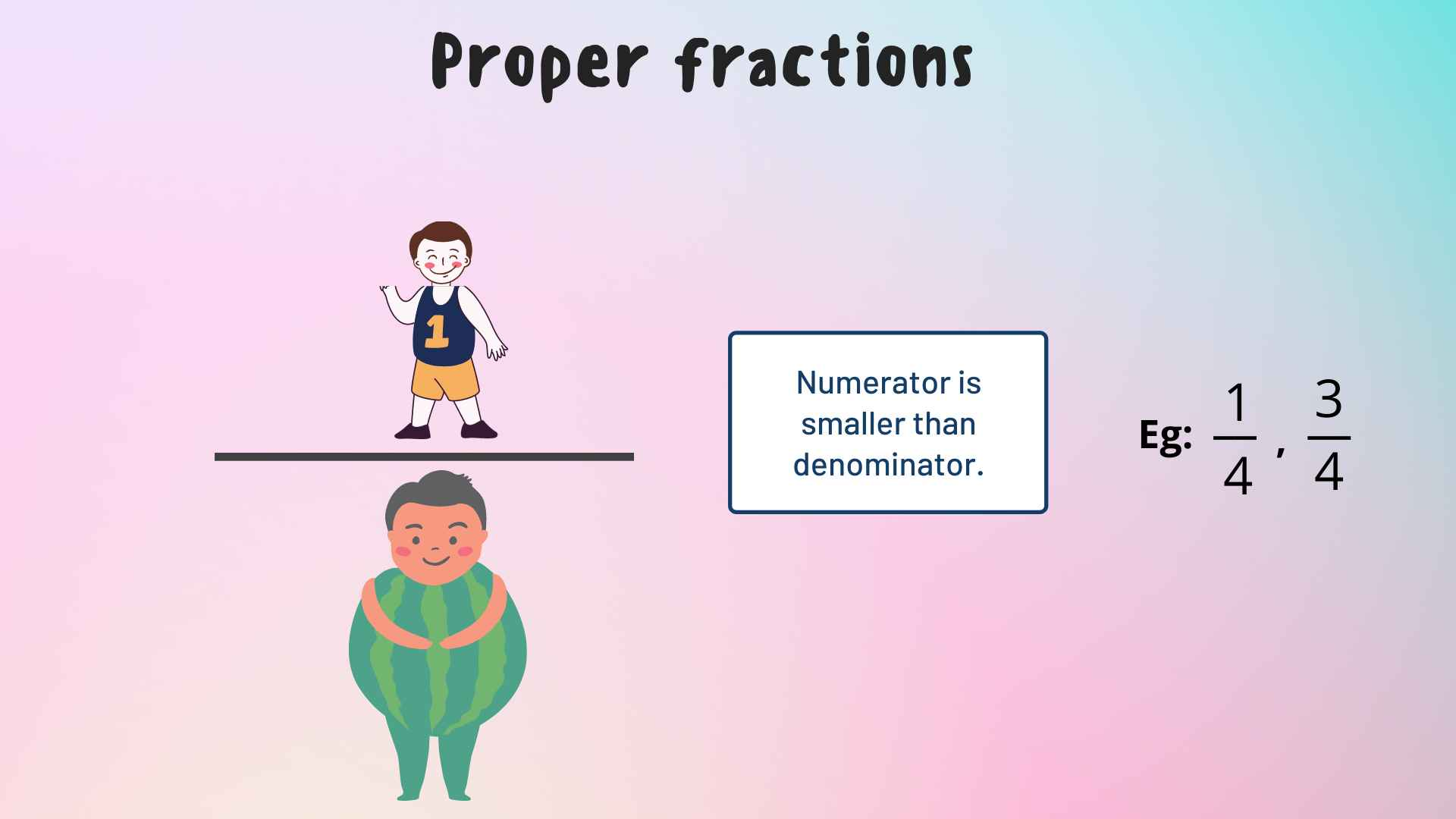The Above Image illustrating Proper Fractions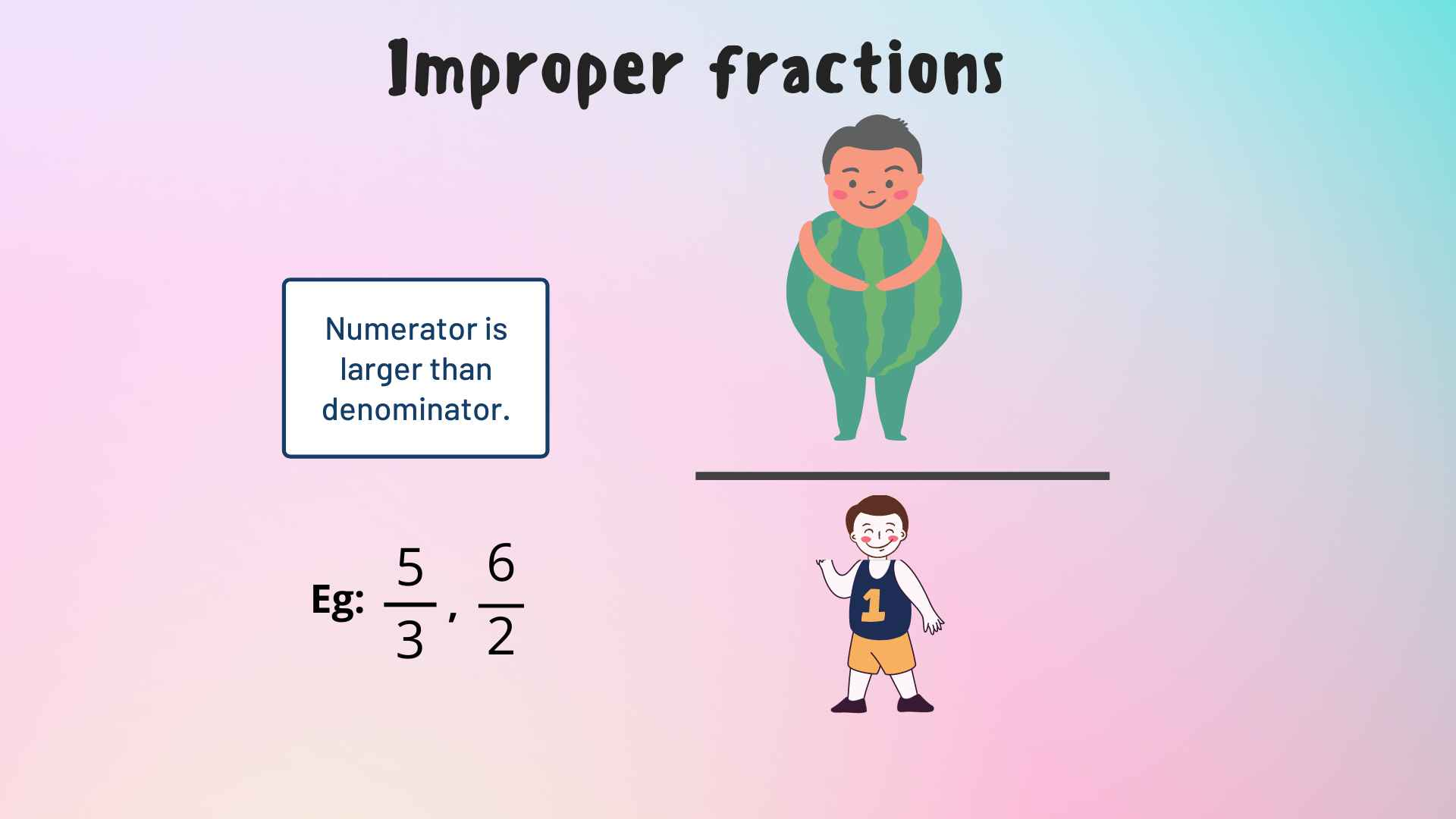The Above Image Illustrates Improper Fractions

The Above Image Illustrates Mixed Fractions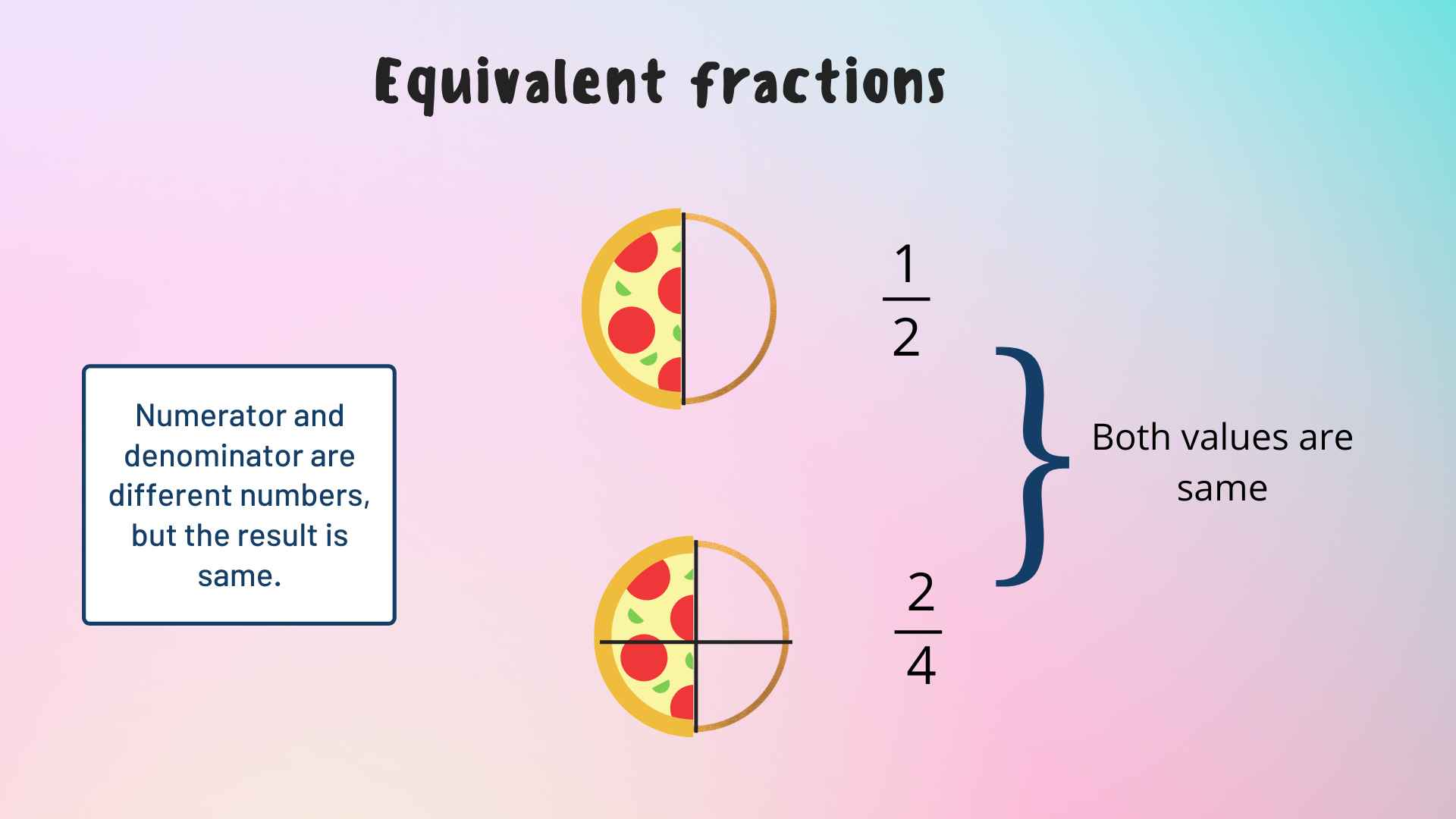The Above Image Illustrates Equivalent Fractions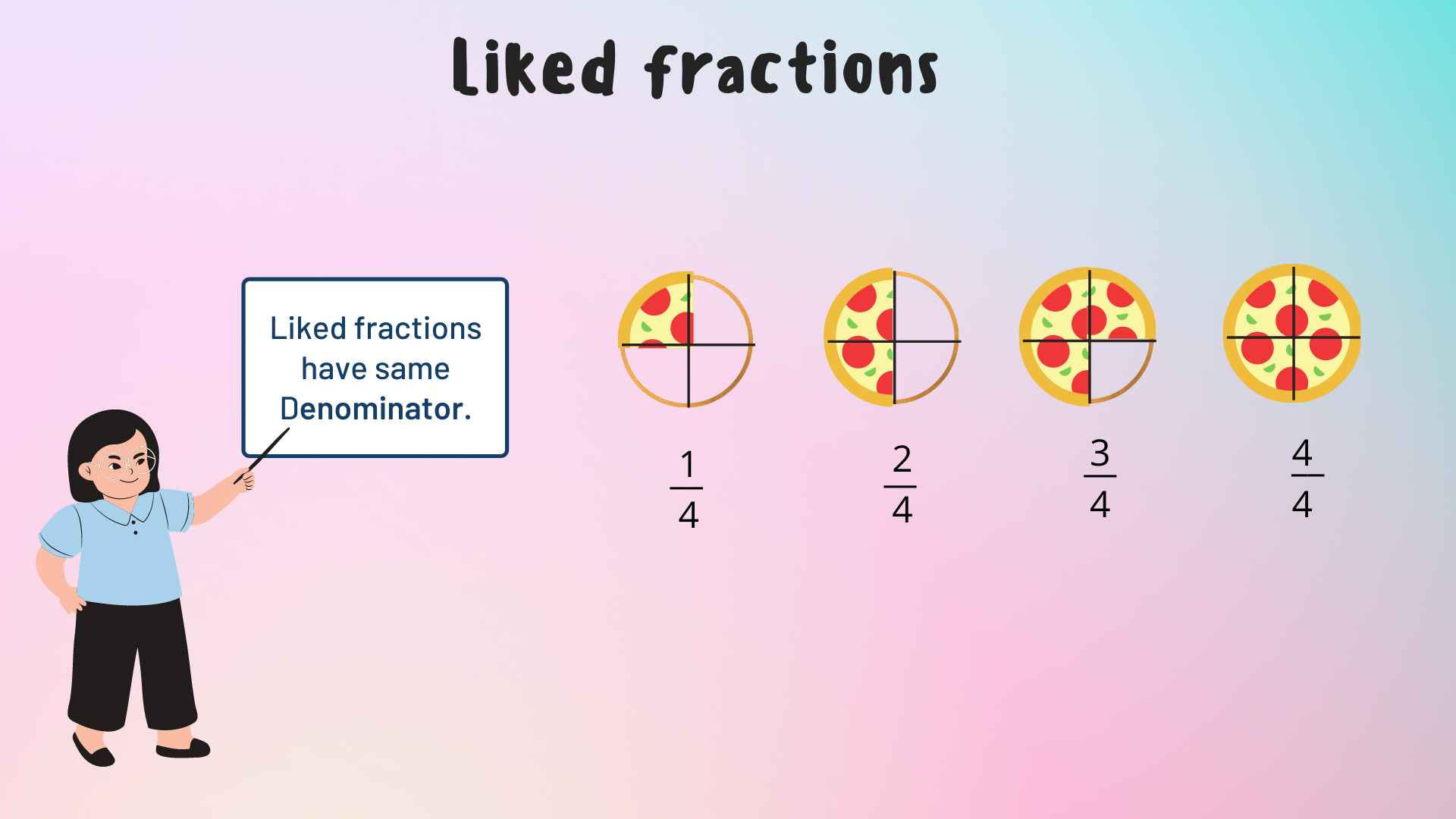The Above Image Illustrates Liked Fractions

## Decimals

The decimal number system is a positional notation that has a base of 10. It refers to numbers with a fractional part separated from an integer part by a decimal separator. Decimals allow us to write fractions without having to write a fraction with a numerator and denominator.

The Above Image Illustrates the Parts of a Decimal Number

## Decimal Place Value Chart

The position of each digit is important when writing any number. When we go to the right in the below place value chart, the value of the digit becomes 10 times smaller, i.e. one-tenth, and when we move to the left, the value of the digit becomes ten times larger.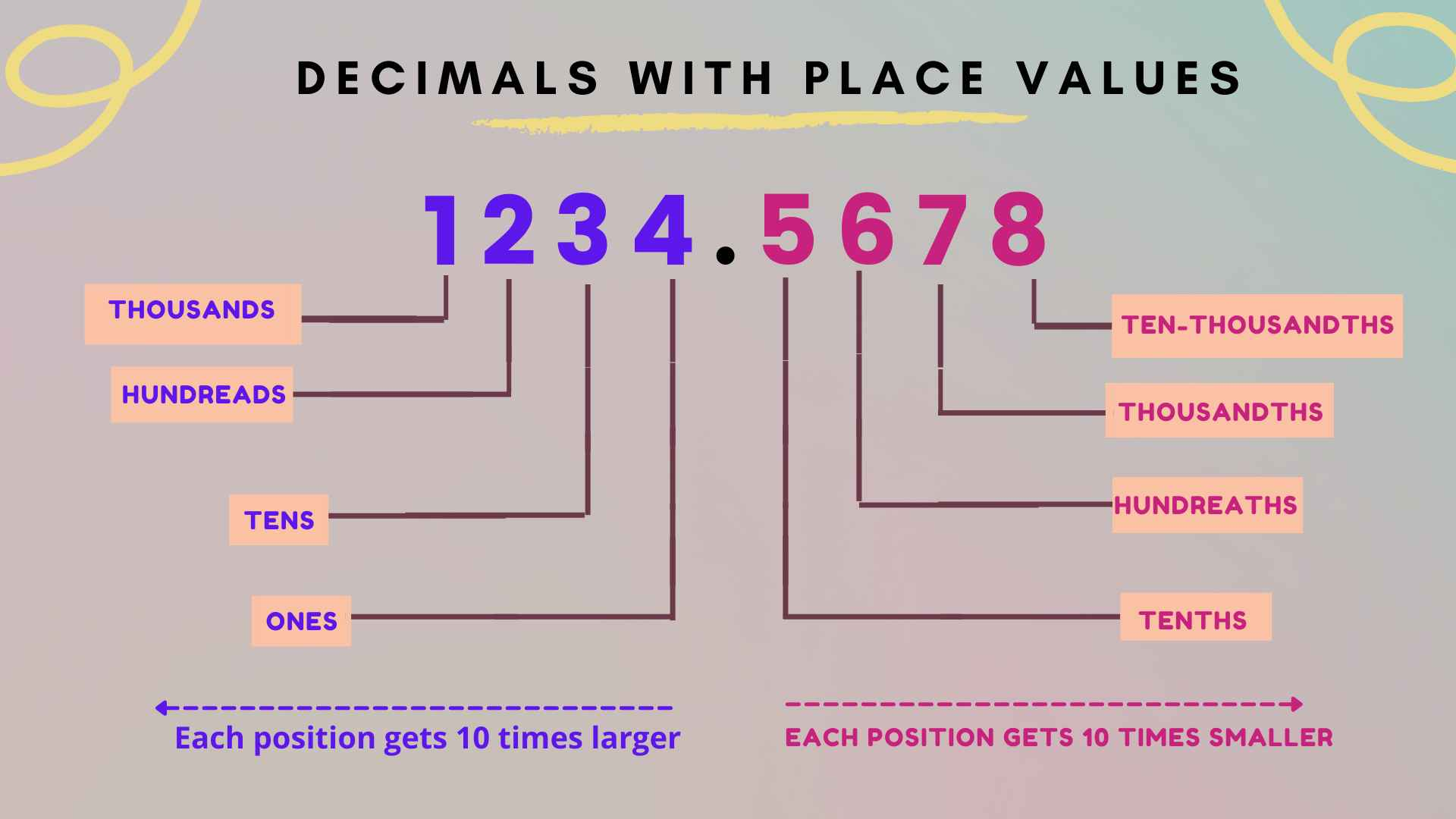The Above Image Illustrates the Decimal Number with Corresponding Place Values

## Fractions to Decimals

Simply divide the numerator by the denominator to convert the fraction to decimal.

4/10, for example, is a fraction. When we split it, we get 0.4.

The Above Image Shows an Activity of Writing a Fraction and Converting it to a Decimal Value

Real-life example to understand fraction to decimal conversion:

Tara divides her A4 Sheet into 12 equal parts. She drew the fruits of different kinds on each part of the Sheet. Amongst the 12 slots, she reserved 5 equal portions for apples, 3 portions for oranges, and 4 for bananas. Let us write the portion given to fruits of different kinds in a fraction as well as in decimal form.

Image Illustrates the Above Real-life Example

Apples are drawn in the 5/12 or 0.4166 part of the sheet.

Oranges are drawn in the 3/12 or 0.25 part of the sheet.

Bananas are also drawn in the 4/12 or 0.333 part of the sheet.

Examples

1. Convert 16/20 into decimal form.

Sol: To convert fraction to decimal, divide 16 by 20, and we get the decimal form.

Thus, 16/20 = 0.8

Hence, the decimal form of 16/20 is 0.8

2. Express 2.25 in fraction form.

Sol: The given decimal number is 2.25

The expanded form of 2.25 is

= 225 x (1/100)

= 225 /100

=9/4

Hence, the equivalent fraction for 2.25 is 9/4.

## Fraction to Decimal Chart

The fraction to decimal chart is an easy way to get the converted numbers. The chart shows the decimal values of the most frequently used fractions. Fractions will be less or greater than one. Fractions less than 1 have a numerator < denominator. They are known as Proper fractions. Proper fractions have decimal values that are always less than 1. Fractions which have numerator ≥ denominator are called improper fractions. They have 1 or more decimal values. Let us see how the numbers would look when we convert fractions to decimal form using the fraction to the decimal chart shown below: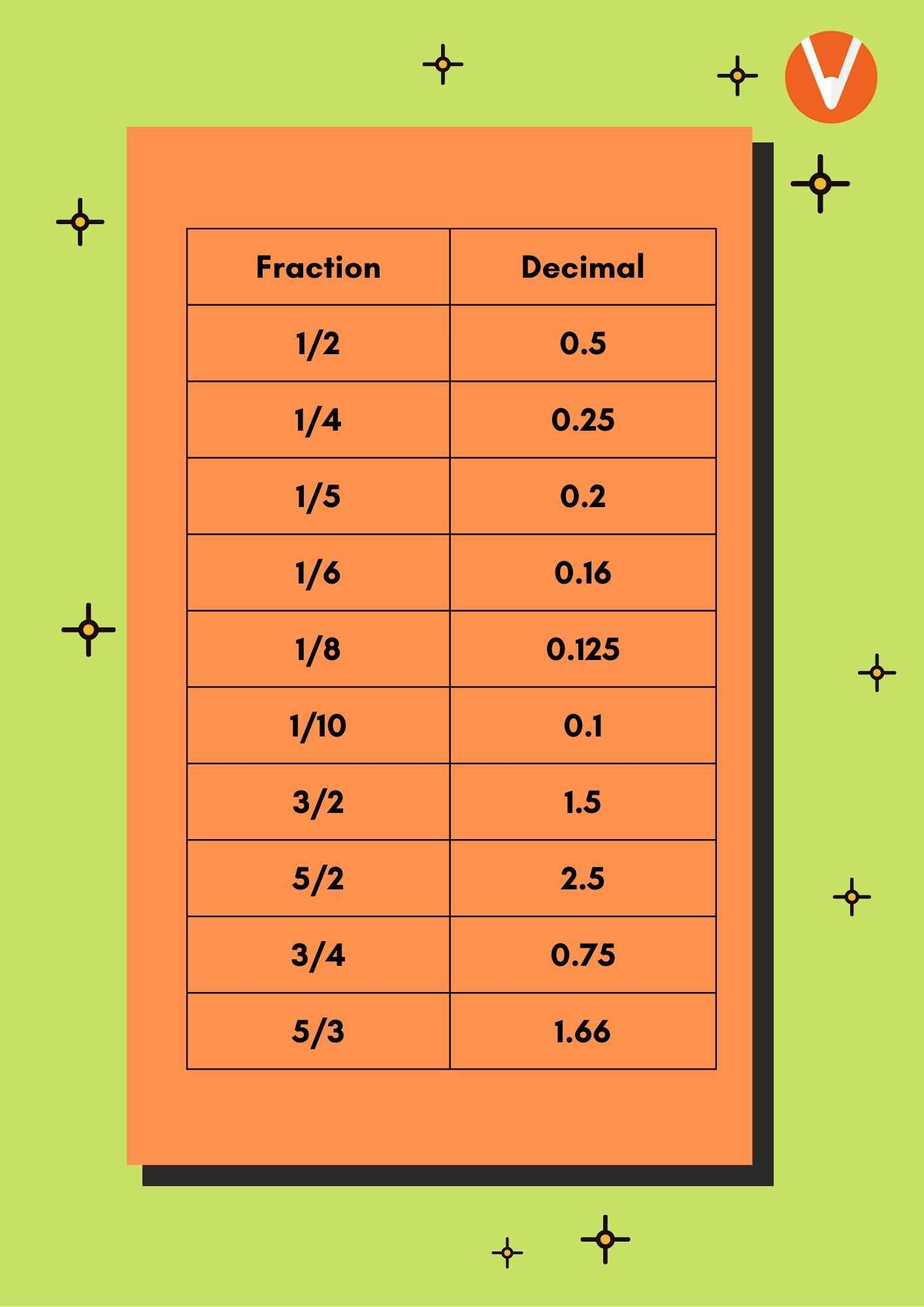The Image Shows the “Fraction to Decimal Chart”

## Conclusion

Both fractions and decimals are used to represent parts of a whole; both fractions and decimals express a number less than one. In order to complete a maths problem, you may need to convert a fraction to a decimal. This concept has been thoroughly covered in this article.

## FAQs on Easy Steps to Convert Fractions into Decimals

1. How to Convert Fractions to Decimals?

Converting fractions to decimals is not a tricky task; but, in order to understand the operations, you must first understand decimal division. Understanding how to deal with terminating and repeating decimals in the final answer is also a crucial skill in this topic. On the other hand, in fractions, the numerator is an integer that comes before or after the slash, and the denominator is an integer that comes after or below the line. The line is commonly used as a dividing sign. To convert a fraction to a decimal, divide the numerator by the denominator.

2. Explain a strategy which will help kids in converting fractions to decimals:

To better understand the idea, follow the following strategy:

• When a fraction contains a denominator of a tenth, hundredth, thousandth, or other value that corresponds to the place value of a decimal, just move the digits in the numerator to the right of the decimal point.

• Divide the numerator by the denominator to convert a fraction that does not have a denominator of tenths, hundredths, thousandths, etc. When using long division, always add zeros as placeholders and round the quotient to the nearest hundredth if the divisor is greater than the dividend.